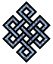#fail2ban bad ip database: ip 219.65.65.195

### | ip database | live view | stats | report | help | api key:

 ip: 219.65.65.195 hostname: 219.65.65.195.STATIC-Banglore.vsnl.net.in country:[IN] India first reported: 20.03.2018 16:35.51 GMT+0200 last reported: 26.11.2018 11:16.32 GMT+0200 time period: 250d 18h 40m 41s total reports: 10 reported by: 4 host(s) filter(s): ssh (9) ssh (1) tor exit node no badips.com db Lookup## port scan of '219.65.65.195':

[-hide]
```# Nmap 6.40 scan initiated Tue Mar 20 16:36:01 2018 as: /usr/bin/nmap -sU -sS -O 219.65.65.195
Nmap scan report for 219.65.65.195.STATIC-Banglore.vsnl.net.in (219.65.65.195)
Host is up (0.14s latency).
Not shown: 1000 open|filtered ports, 987 filtered ports
PORT      STATE  SERVICE
22/tcp    open   ssh
80/tcp    open   http
443/tcp   open   https
1000/tcp  open   cadlock
3000/tcp  open   ppp
3306/tcp  closed mysql
5000/tcp  open   upnp
5002/tcp  open   rfe
5901/tcp  open   vnc-1
7000/tcp  open   afs3-fileserver
8080/tcp  closed http-proxy
9090/tcp  closed zeus-admin
48080/tcp closed unknown
No exact OS matches for host (If you know what OS is running on it, see http://nmap.org/submit/ ).
TCP/IP fingerprint:
OS:SCAN(V=6.40%E=4%D=3/20%OT=22%CT=3306%CU=%PV=N%G=Y%TM=5AB12A94%P=x86_64-p
OS:c-linux-gnu)SEQ(SP=109%GCD=1%ISR=10C%TI=Z%TS=A)OPS(O1=M5B4ST11NW7%O2=M5B
OS:4ST11NW7%O3=M5B4NNT11NW7%O4=M5B4ST11NW7%O5=M5B4ST11NW7%O6=M5B4ST11)WIN(W
OS:1=7120%W2=7120%W3=7120%W4=7120%W5=7120%W6=7120)ECN(R=Y%DF=Y%TG=40%W=7210
OS:%O=M5B4NNSNW7%CC=Y%Q=)T1(R=Y%DF=Y%TG=40%S=O%A=S+%F=AS%RD=0%Q=)T2(R=N)T3(
OS:R=N)T4(R=N)T5(R=Y%DF=Y%TG=40%W=0%S=Z%A=S+%F=AR%O=%RD=0%Q=)T6(R=N)T7(R=N)
OS:U1(R=N)IE(R=N)

OS detection performed. Please report any incorrect results at http://nmap.org/submit/ .
# Nmap done at Tue Mar 20 16:36:52 2018 -- 1 IP address (1 host up) scanned in 51.84 seconds
```
```Σ = 57 | Δt = 0.0076231956481934s
```